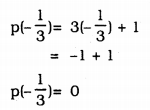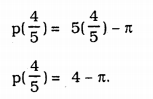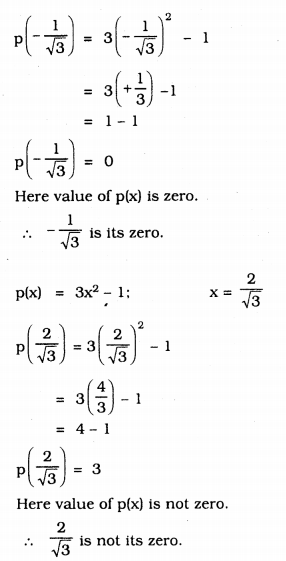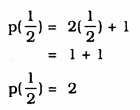# KSEEB Solutions for Class 9 Maths Chapter 4 Polynomials Ex 4.2

In this chapter, we provide KSEEB SSLC Class 9 Maths Chapter 4 Polynomials Ex 4.2 for English medium students, Which will very helpful for every student in their exams. Students can download the latest KSEEB SSLC Class 9 Maths Chapter 4 Polynomials Ex 4.2 pdf, free KSEEB SSLC Class 9 Maths Chapter 4 Polynomials Ex 4.2 pdf download. Now you will get step by step solution to each question.

## Karnataka Board Class 9 Maths Chapter 4 Polynomials Ex 4.2

KSEEB Solutions For Class 9 Maths Polynomials Question 1.
Find the value of the polynomial 5x – 4x2 + 3 at
i) x = 0
ii) x = -1
iii) x = 2
i) f(x) = 5x – 4x2 + 3 x = 0 then,
f(0) = 5(0) – 4(0)2 + 3
=0 – 0 + 3
f(0) = 3

ii) f(x) = 5x – 4x2 + 3 x = -1 then,
f(-1) = 5(-1) – 4(-1)2 + 3
= -5 – 4(+1) + 3
= -5 – 4 + 3
= -9 + 3
f(-1) = -6

iii) f(x) = 5x – 4x2 + 3 x = 2 then,
f(2) = 5(2) – 4(2)2 + 3
= 5(2) – 4(4) + 3
= 10 – 16 + 3
= 13 – 16
f(2) = -3

9th Maths Polynomials Exercise 4.2 Question 2.
Find p(0), p(1) and p(2) for each of the following polynomials :
i) p(y) = y2 – y + 1
ii) p(t) = 2 + t + 2t2 – t3
iii) p(x) = x3
iv) p(x) = (x – 1) (x + 1)
i) (a) p(y) = y2 – y + 1
p(0) = (0)2 – 0 + 1
= 0 – 0 + 1
∴ p(0) = =1

(b) p(y) = y2 – y + 1
p(1) = (1)2 – 1 + 1
= 1 – 1 + 1
∴ p(1) = 1

(c) p(y) = y2 – y +
p(2) = (2)2 – 2 + 1
= 4 – 2 + 1
= 5 – 2
∴ p(2) = 3

ii) (a) p(t) = 2 + t + 2t2 – t3
p(0) = 2 + 0 + 22 – (0)3
= 2 + 0 + 0 – 0
∴ p(0) = 2

(b) p(t) = 2 + t + 2t2 – t3
p(1) = 2 + 1 + 2(1)2 – (1)3
= 2 + 1 + 2(1) – 1
= 2 + 1 + 2 – 1
∴ p(1) = 4

(c) p(t) – 2 + t + 2t2 – t3
p(2) = 2 + 2 + 2(2)2 – (2)3
= 2 + 2 + 2(4) – 8
= 2 + 2 + 8 – 8
∴ p(2) = 4

iii) (a) p(x) = x3
p(0) = (0)3
p(0) = 0

(b) p(x) = x3
p(1) = (1)3
∴ p(1) = 1

(c) p(x) = x3
p(2) = (2)3
∴ p(2) = 8

iv) (a) p(x) = (x – 1)(x + 1)
p(x) = x2 – 1 [∵ (a + b)(a – b) = a2 – b]
p(0) = (0)2 – 1
= 0 – 1
∴ p(o) = -1

(b) p(x) = (x – 1)(x + 1)
p(x) = x2 – a [∵ (a + b)(a – b) = a2 – b2]
p(1) = (1)2 – 1
= 1 – 1
∴ p(1) = 0

(c) p(x) = (x – 1)(x + 1)
p(x) = x2 – 1 [∵ (a + b)(a – b) = a2 – b2]
p(2) = (2)2 – 1
= 4 – 1
∴ p(0) = 3

KSEEB Solutions For Class 9 Maths Polynomials Exercise 4.2 Question 3.
Verify whether the following are zeroes of the polynomial, induced against them.
i) p(x) = 3x + 1; x=−13
ii) p(x) = 5x – π; x=45
iii) p(x) = x2 – 1; x = 1, -1
iv) p(x) = (x + 1) (x – 2); x = -1, 2
v) p(x) = x2; x = 0
vi) p(x) = lx + m; x=−ml
vii) p(x) = 3x2 – 1; x=−13√,23√
viii) p(x) = 2x + 1; x=12
i) p(x) = 3x + 1; x=−13Here value of polynomial is zero.
x=−13 is not zero of the polynomial

ii) p(x) = 5x – π; x=45Here value of polynomial is not zero.
x=45 is not zero of the polynomial

iii) p(x) = x2 – 1; x = 1, -1
p(1) = (1)2 – 1
= 1 – 1
p(1) = 0
Here value of p(x) is zero.
hence its zero is 1.
p(x) = x2 – 1; x = -1
p(-1) = (-1)2 – 1
= 1 – 1
p(-1) = 0
Here value of p(x) is zero.
∴ -1 is zero.

iv) p(x) = (x – 1)(x – 2); x = -1, 2
p(x) = x2 – 2x + x – 2
p(x) = x2 – x + 2 x = -1
p(-1) = (-1)2 – (-1)2 + 2
= 1 + 1 + 2
p(-1) = 4
Here value of polynomila is not zero.
∴ -1 is not zero.
p(x) = x2 – x + 2 x = 2
p(2) = (2)2 – (2) + 2
= 4 – 2 + 2
p(-1) = 4
Here value of polynomial is not zero.
∴ 2 is not zero.

(v) p(x) = x2; x = 0
p(0) = (0)2
p(0) = 0
Here value of p(x) is zero.
∴ 0 is its zero.

vi) p(x) = lx + m; x=−ml
p(−ml)=l(−ml)+m
= -m + m
= 0
Here p(x) is zero.
∴ −ml is its zero.

(vii) p(x) = 3x2 – 1; x=−13√,23√viii) p(x) = 2x + 1; x=12Here value of p(x) is not zero.
∴ 12 is not its zero.

Polynomials Class 9 Exercise 4.2 Solutions Question 4.
Find the zero of the polynomial in each of the following cases :
(i) p(x) = x + 5
(ii) p(x) = x – 5
(iii) p(x) = 2x + 5
(iv) p(x) = 3x – 2
(v) p(x) = 3x
(vi) p(x) = ax, a ≠ 0
(vii) p(x) = cx + d, c ≠ 0, c, d are real numbers.
i) p(x) = x + 5
Let p(x) =0, then,
p(x) = x + 5 = 0
x = 0 – 5
∴ x = -5
-5 is zero of p(x).

ii) p(x) = x – 5
If p(x) = 0, then
p(x) = x – 5 = 0
x = 0 + 5
∴ x = 5
5 is the zero of p(x).

iii) p(x) = 2x + 5
If p(x)= 0, then
p(x) = 2x + 5 = 0
2x = – 5
∴ x=−52
−52 is the zero of p(x).

iv) p(x) = 3x – 2
If p(x)= 0, then
p(x) = 3x – 2 = 0
3x = 2
∴ x=23
23 is the zero of p(x).

v) p(x) = 3x
If p(x) = 0, then
p(x) = 3x = 0
∴ x=03
03 is the zero of p(x)

vi) p(x) = ax, a ≠ 0
If p(x)= 0, then
p(x) = ax = 0
∴ x=0a ∴ x = ∞(infinity)
∞ is the zero of p(x).

vii) p(x) = cx + d, c ≠ 0, c, d are real numbers
If p(x)= 0, then
p(x) = cx + d = 0
cx = 0 – d
cx = -d
∴ x=−dc
−dc is the zero of p(x).

All Chapter KSEEB Solutions For Class 9 Maths

—————————————————————————–

All Subject KSEEB Solutions For Class 9

*************************************************

I think you got complete solutions for this chapter. If You have any queries regarding this chapter, please comment on the below section our subject teacher will answer you. We tried our best to give complete solutions so you got good marks in your exam.

If these solutions have helped you, you can also share kseebsolutionsfor.com to your friends.

Best of Luck!!